## Example Questions

### Example Question #13 : Percentage

The girl's tennis team decided to do multiple fundraisers to raise enough to buy new equipment for next season. The following is a table of 4 of the fundraisers they did and the fraction of the total funds each raised.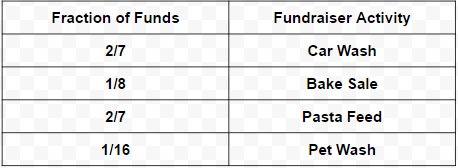The last fundraiser they did earned them the final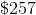they needed. What percent of the total funds raised did the last fundraiser raise?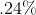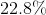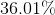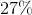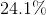Explanation:

The question asks to find what percent the last fundraiser makes up of the total funds raised. The information that's given is how much that last fundraiser made and what fraction the other fundraisers made.

This problem may be solved without the amount of money the last fundraiser raised. The reason is that we know that only 5 fundraisers were done. We're given what the fraction of the other fundraisers were.

If we were to add all the fractions together, including the last fundraiser, the sum of all would equal 1.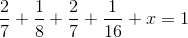Fractions are another version of decimals but also another version of percentages. Percentages are essentially a prettier way of showing parts (or fractions) of a whole, which would be 100%. In the case of fractions, this is the same logic, which is why when all the fractions are summed, they equal 1. This 1 represents 100%.
Upon using lowest common denominators, adding the fractions together, and solving for x, x is calculated to be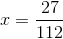We've almost obtained the final answer. Now it's just a matter of converting this fraction into a percent. As mentioned previously, fractions are another version of decimals. Think of decimals as being the middle conversion between fractions and percents. All that needs to be done to a decimal to turn it in to a percent is to multiply it by 100. For instance, .50 becomes 50%. Conversely, .50 is the same asin fraction form. Therefore, one half is 50%.
In order to solve for x in percent, the same conversion must be done.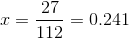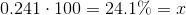### Example Question #1 : How To Find Fractional Percentages

Convert the following to a percentage.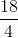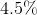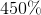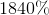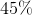Explanation:

To convert a fraction into a percentage, simply simplify the fraction into a decimal and multiply by 100.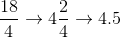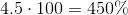### Example Question #15 : Percentage

If a dress in a store originally costs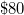, what does it cost (in dollars) after a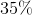discount is applied?

$28$45
$52$70
$63 Correct answer:$52
To findof 80,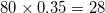. Next, subtract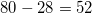, for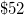as the final answer.